# Element Table 可以实现常见的有用的功能介绍

/

### 1.行背景色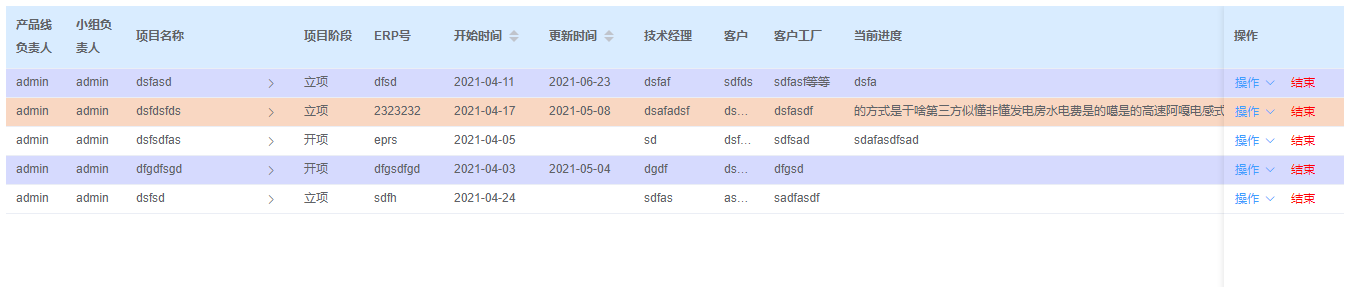table 组件提供了row-style 属性，说明：行的 style 的回调方法，也可以使用一个固定的 Object 为所有行设置一样的 Style。

setRowStyle({row, rowIndex}) {      return {        'background-color': this.rowsBgColor[rowIndex]      } }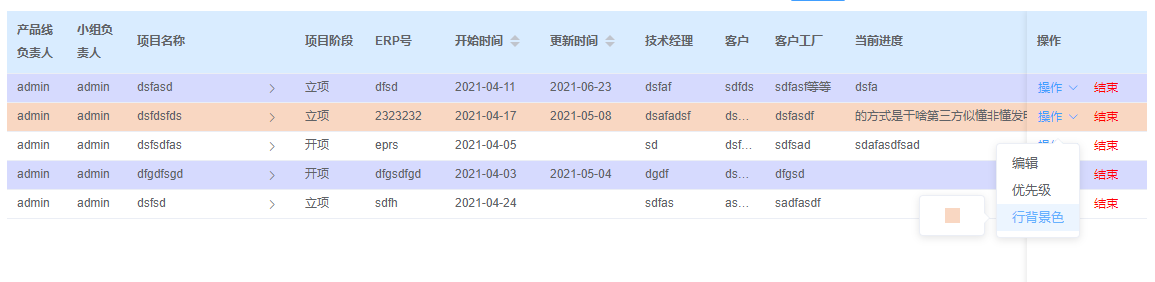### 2.合并单元格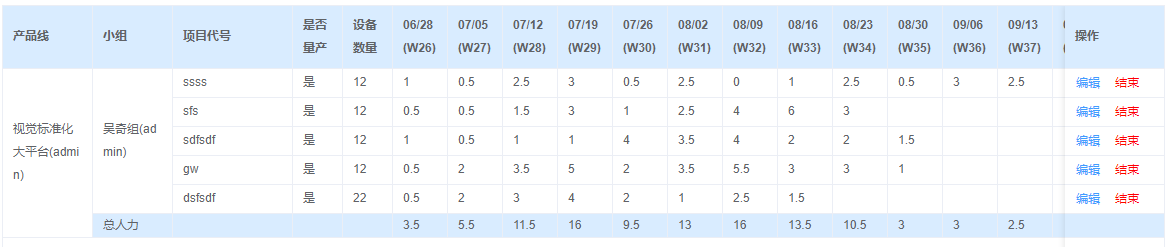Table 组件下面有 span-method 属性，说明：合并行或列的计算方法，传入的参数有 row, column, rowIndex, columnIndex。

:span-method="objectSpanMethod"

objectSpanMethod ({ row, column, rowIndex, columnIndex }) {      if (columnIndex === 0 || columnIndex === 1) {        const _rowspan = this.afterSpanArray[column.property][rowIndex]        const _colspan = _rowspan > 0 ? 1 : 0        return {          rowspan: _rowspan,          colspan: _colspan        }      } }

3.表格统计
Table 组件提供了 show-summary 属性，说明：是否在表尾显示合计行，它的值是 Boolean 类型的，如果设置为 True，则表格底部为会增加“合计”的一行，它只会统计单元格值是数字的列。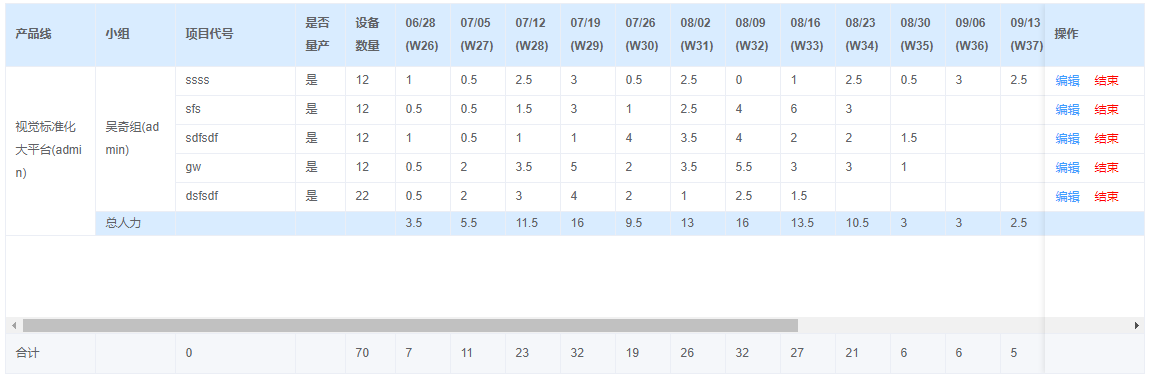:summary-method="getSummaries"

getSummaries(param) {      const { columns } = param      const sums = []      const weekSum = {}      this.deptManpowerSum.forEach((deptManpower, index) => {        let key = 'col' + deptManpower.week.replace(/-/g, '')        weekSum[key] = deptManpower.weeklyTotal      })      columns.forEach((column, index) => {        if (index === 0) {          sums[index] = '部门总人力'          return        }        if (weekSum.hasOwnProperty(column.property)) {          sums[index] = weekSum[column.property]        } else {          sums[index] = ''        }      })      return sums }

### 4.行展开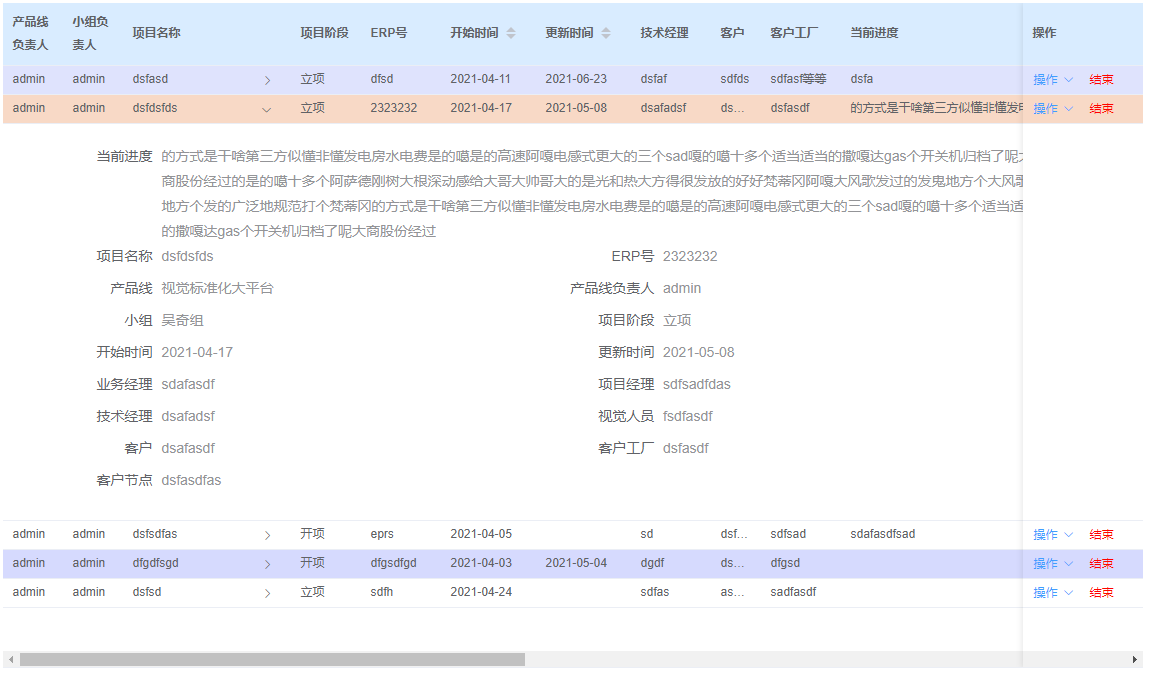:row-key="(row) => {return row.id}":expand-row-keys="expandRows"

<el-table-column type="expand">    <template v-slot="{row}"></template></el-table-column>

@row-click="clickRowHandle"

clickRowHandle(row) {  if (this.expandRows.includes(row.id)) {    this.expandRows = this.expandRows.filter(val => val !== row.id)  } else {    this.expandRows.push(row.id)  }}

@expand-change="rowExpandChange"

rowExpandChange(row, expandedRows) {  this.clickRowHandle(row)}